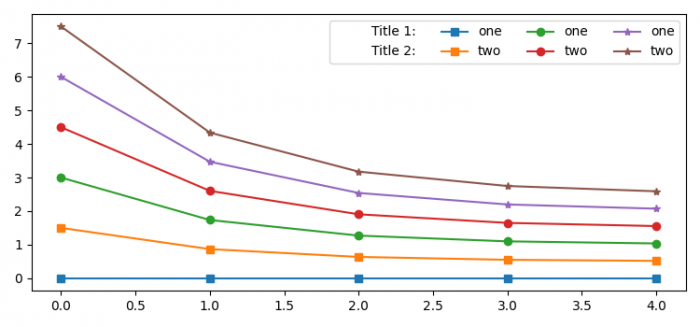# How to add titles to the legend rows in Matplotlib?

MatplotlibPythonData Visualization

To add titles to the legend rows in Matplotlib, we can take the following steps −

• Set the figure size and adjust the padding between and around the subplots.

• Create y data points using numpy.

• Make lists of markers and labels.

• Create a figure and a set of subplots.

• Plot the lines using plot() method, with different labels and markers.

• Get the plot handlers for half of the plot.

• Get the labels for the legends.

• Place the legends on the plot.

• To display the figure, use show() method.

## Example

import matplotlib.pyplot as plt
import numpy as np

plt.rcParams["figure.figsize"] = [7.50, 3.50]
plt.rcParams["figure.autolayout"] = True

y = np.exp(-np.arange(5))

markers = ["s", "o", "*"]
labels = ["one", "two"]

fig, ax = plt.subplots()

for i in range(6):
ax.plot(y * i + i / 2., marker=markers[i // 2], label=labels[i % 2])
h, l = ax.get_legend_handles_labels()
ph = [plt.plot([], marker="", ls="")] * 2
handles = ph + h

labels = ["Title 1:", "Title 2:"] + l
plt.legend(handles, labels, ncol=4)

plt.show()

## Output